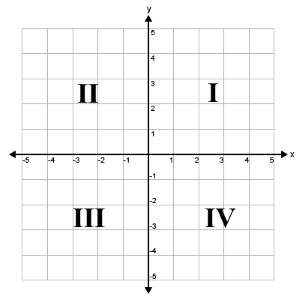• ### Browse All Lessons

##### Assign Lesson

Help Teaching subscribers can assign lessons to their students to review online!

 Tweet# Quadrants of the Coordinate PlaneIntroduction: A coordinate plane is two perpendicular number lines used to graph pairs of numbers. The horizontal line is the x-axis. Positive numbers are located to the right of zero and negative number are located left of zero. The vertical line is the y-axis. Positive number are located above zero and negative numbers are located below zero. The lines intersect at each line's zero and divide the plane into four sections.

The four sections of the coordinate plane are quadrants. The four quadrants are labeled counterclockwise using Roman numerals, starting in the upper right section of the graph.

Quadrant I is the first quadrant. It is located on the upper right side of the plane. All points plotted in the first quadrant have both positive x-coordinates and positive y-coordinates

Quadrant II is the second quadrant. It is located on the upper left side of the plane. All points plotted in the second quadrant have negative x-coordinates and positive y-coordinates.

Quadrant III is the third quadrant. It is located on the lower left side of the plane. All points plotted in the third quadrant have both negative x-coordinates and negative y-coordinates.

Quadrant IV is the fourth quadrant. It is located on the lower right side of the plane. All points plotted in the fourth quadrant have positive x-coordinates and negative y-coordinates.

The table summarizes the four quadrants of the coordinate plane.
IPositivePositive(3, 5)
IINegativePositive(-3, 5)
IIINegativeNegative(-3, -5)
IVPositiveNegative(3, -5)

Directions for This Lesson: In this lesson, you will learn about the four quadrants of the coordinate plane. First, try the practice questions to determine what you already know. Then, watch the video lesson to learn more.

Required Video:

Related Worksheets:

Related Lessons: